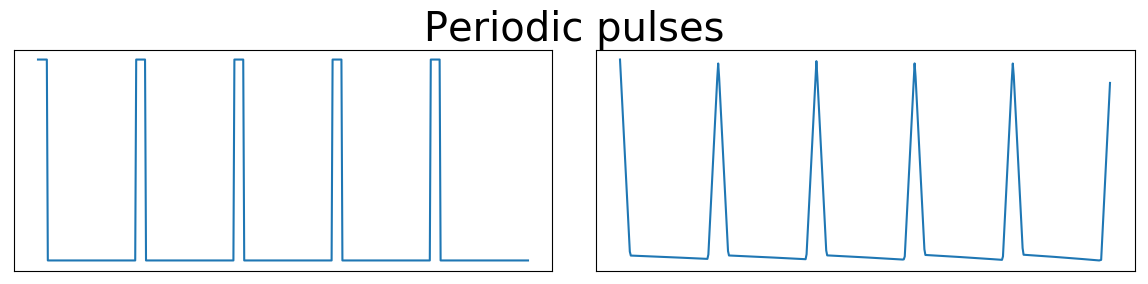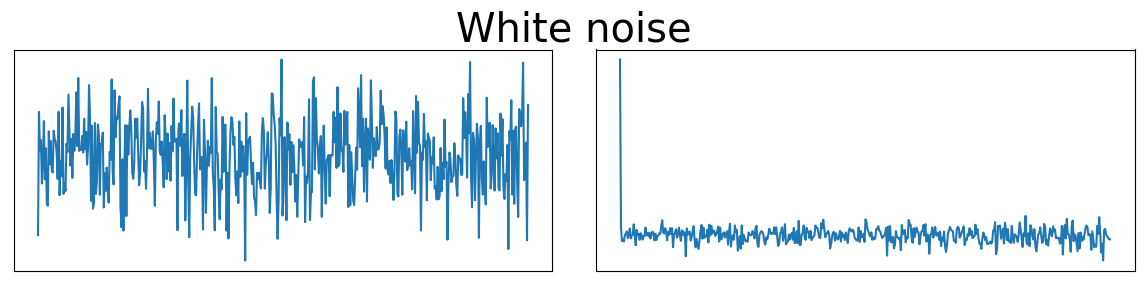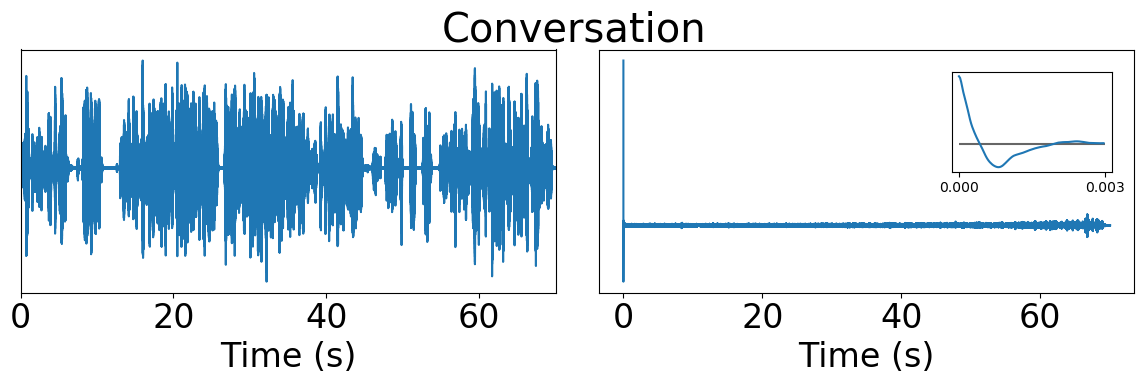# Autocorrelation Sonification

## How does autocorrelation functions sounds like?

Ansatz
, in 06 June 2017
autocorrelation

# Correlation with it self¶

The autocorrelation function of a time series computes the correlation with it self at different time lags. For a real finite and discrete signal $X_n$ we have the unbiased autocorrelation $R_{XX}$ defined like:

$\displaystyle R_{XX}(l)=\frac{1}{N-l}\sum _{n}^N X_n X_{n-l}.$

Some basic examples shows that the autocorrelation computation preserves periodic information and forgets uncorrelated randomness.## Voices¶

Lets look how are the autocorrelations of more natural signals, and how they sound. First a fragment of a man and woman having a conversation. The autocorrelation shows that the signal is uncorrelated in the long term, but has some positive and negative correlation in the short scale, between 0 and 2 ms (inset plot). Lets hear what this signal sounds like. The first 100 ms sound like an impulse, and it encodes the some average timbre characteristics of the original voices. The rest of the autocorrelation sounds like a room full of people chatting. We could think this process as a convolution reverb with made with a room impulse response that is the original sound it self.Original